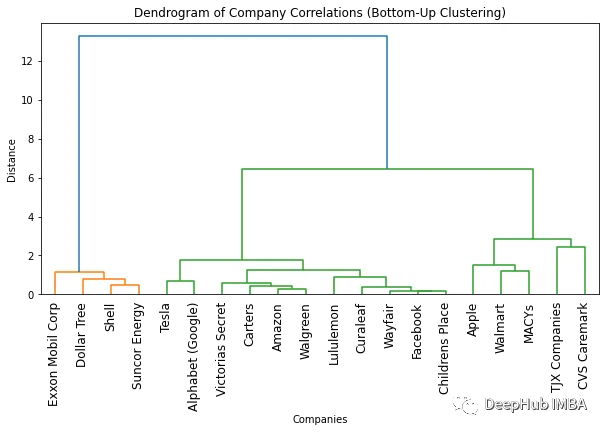0

# 使用树状图可视化聚类

## 树状图

“树状图”一词来自希腊语“dendron”（树）和“gramma”（绘图）。1901年，英国数学家和统计学家卡尔皮尔逊用树状图来显示不同植物种类之间的关系。他称这个图为“聚类图”。这可以被认为是树状图的首次使用。

## 数据准备

`````` importpandasaspd
importrequests

'Exxon Mobil Corp':'XOM','Lululemon':'LULU','Walmart':'WMT','Carters':'CRI','Childrens Place':'PLCE','TJX Companies':'TJX',
'Victorias Secret':'VSCO','MACYs':'M','Wayfair':'W','Dollar Tree':'DLTR','CVS Caremark':'CVS','Walgreen':'WBA','Curaleaf':'CURLF'}
``````

`````` importtime

all_data={}
forkey,valueincompanies.items():
# Replace YOUR_API_KEY with your Alpha Vantage API key
response=requests.get(url)
data=response.json()
time.sleep(15)
if'Time Series (Daily)'indataanddata['Time Series (Daily)']:
df=pd.DataFrame.from_dict(data['Time Series (Daily)'], orient='index')
else:
print("Time series data is empty or not available.")
df.rename(columns= {'1. open':key}, inplace=True)
all_data[key]=df[key]
``````

`````` # find common dates among all data frames
common_dates=None
fordf_key, dfinall_data.items():
ifcommon_datesisNone:
common_dates=set(df.index)
else:
common_dates=common_dates.intersection(df.index)

common_dates=sorted(list(common_dates))

# create new data frame with common dates as index
df_combined=pd.DataFrame(index=common_dates)

# reindex each data frame with common dates and concatenate horizontally
fordf_key, dfinall_data.items():
df_combined=pd.concat([df_combined, df.reindex(common_dates)], axis=1)

``````

## 层次聚类

1、自上而下聚类

`````` importnumpyasnp
importscipy.cluster.hierarchyassch
importmatplotlib.pyplotasplt

# Convert correlation matrix to distance matrix
dist_mat=1-df_combined.corr()

# Perform top-down clustering
cuts=sch.cut_tree(clustering, n_clusters=[3, 4])

# Plot dendrogram
plt.figure(figsize=(10, 5))
sch.dendrogram(clustering, labels=list(df_combined.columns), leaf_rotation=90)
plt.title('Dendrogram of Company Correlations (Top-Down Clustering)')
plt.xlabel('Companies')
plt.ylabel('Distance')
plt.show()
``````2、自下而上聚类

`````` importnumpyasnp
importscipy.cluster.hierarchyassch
importmatplotlib.pyplotasplt

# Convert correlation matrix to distance matrix
dist_mat=1-df_combined.corr()

# Perform bottom-up clustering

# Plot dendrogram
plt.figure(figsize=(10, 5))
sch.dendrogram(clustering, labels=list(df_combined.columns), leaf_rotation=90)
plt.title('Dendrogram of Company Correlations (Bottom-Up Clustering)')
plt.xlabel('Companies')
plt.ylabel('Distance')
plt.show()
``````## 总结

### “使用树状图可视化聚类”的评论:

##### 关于作者##### Deephub